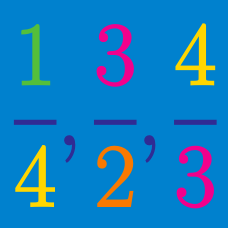Number Theory

# Rational Numbers: Level 4 Challenges

Find the smallest positive integer whose square consists of 5 identical leading digits.

As an explicit example, $$149^2 = 22201$$ would be the answer for 3 identical leading digits.

You have to place dots along a strip such that:

• The first two dots are in the different halves of the strip
• The first three dots are in the different thirds of the strip
• The first four dots are in the different fourths of the strip, and so on.

What is the maximum number of dots you can place on the strip?

Let $$f$$ be a function defined along the rational numbers such that $$f(\tfrac mn)=\tfrac1n$$ for all relatively prime positive integers $$m$$ and $$n$$. The product of all rational numbers $$0<x<1$$ such that $f\left(\dfrac{x-f(x)}{1-f(x)}\right)=f(x)+\dfrac9{52}$ can be written in the form $$\tfrac pq$$ for positive relatively prime integers $$p$$ and $$q$$. Find $$p+q$$.

Let $$\displaystyle \frac ab$$ be the smallest of the fractions that are greater than $$\displaystyle \frac {13}{15}$$ where $$a,b < 500$$ are positive integers.

What is the value of $$a+b$$?

Let $$a_k$$ represent the repeating decimal $$0.\overline{133}_k$$ for $$k \geq 4$$. The product $$a_4 a_5 \cdots a_{99}$$ can be expressed as $$\frac{m}{n!}$$ where $$m, n$$ are positive integers and $$n$$ is as small as possible. $$\frac{m}{n}$$ can be expressed as $$\frac{p}{q}$$ where $$p, q$$ are coprime integers. What is $$p+q$$?

Note: $$0.\overline{133}_k$$ refers to the repeating decimal $$0.133133133\ldots$$ evalauted in base $$k$$.

×

Problem Loading...

Note Loading...

Set Loading...